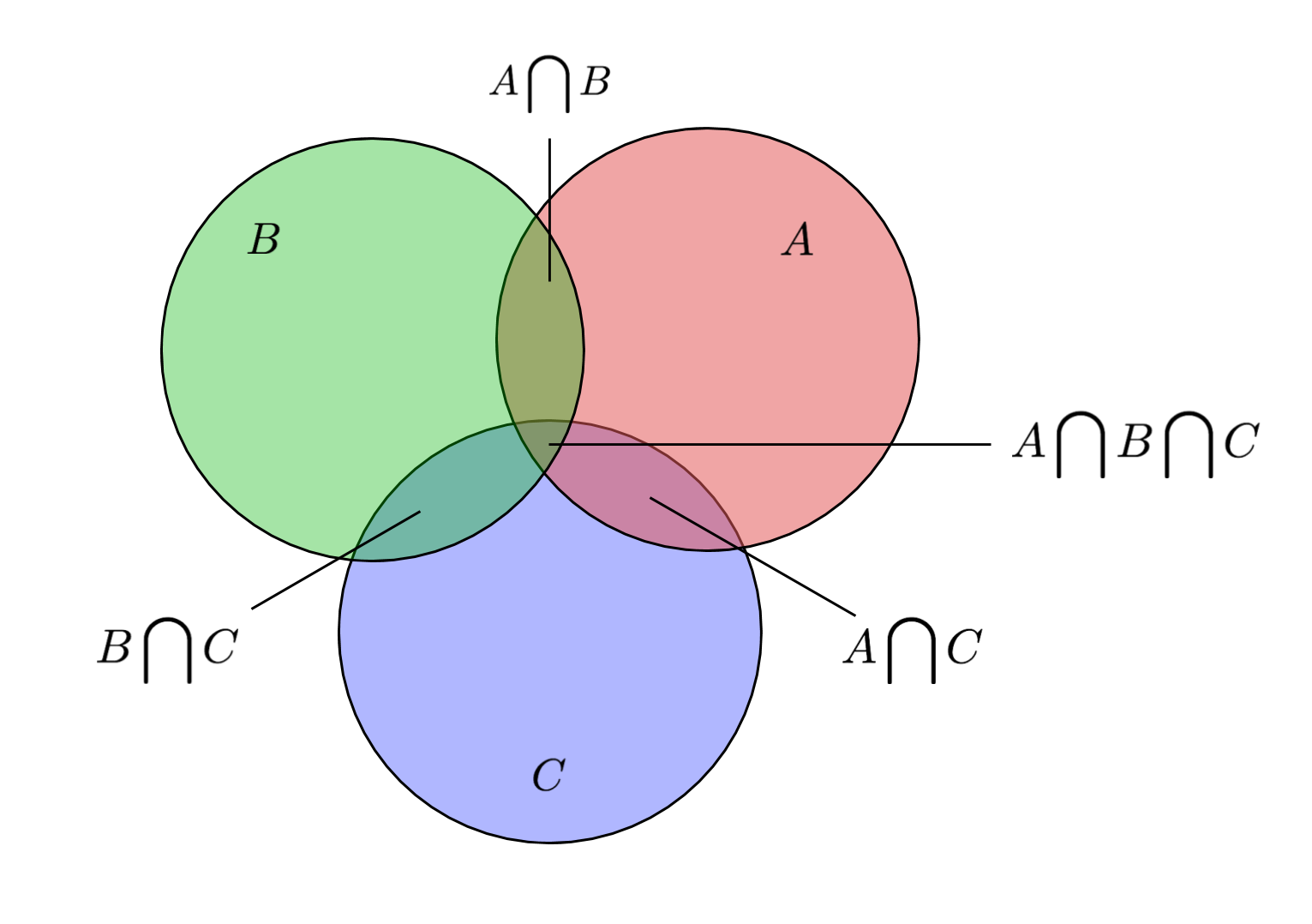# Project Euler Problem 1

Posted in Mathematics

# Overview: The Problem

Project Euler is a website that provides mathematically-oriented programming problems. There are many (over 500) and they are a rich source of profound mathematical insights.

I have been considering a writeup that goes deep into a particular problem, so why not do it with problem 1?

Problem 1 of Project Euler asks:

Find the sum of all the multiples of 3 or 5 below 1000.

It is a pretty simple task - one of the first things covered in a decent programming course is the assortment of mathematical operators, including the modular operator and multiplication operator, useful here.

This problem is also a familiar problem in another guise - any computer science student who has solved a variant of the fizz buzz problem will recognize this task (in the fizz buzz problem, you print fizz every time a number is divisible by 3 and buzz every time it is divisible by 5, etc.)

It is a deceptively simple problem. In fact, it is so easy to solve with a computer that you almost lose a sense of what the manual process would look like. How might we perform this task by hand?

Finally, it is an example of a problem in which we are trying to find the number of outcomes of several classes of events, and some of the events are labeled with both classes. This means it will be important to learn and apply the Inclusion-Exclusion Principle. (Fortunately, this principle is fairly straightforward to apply.)

We will get to the algorithm to counting these factors by hand, and handling more complicated constraints as well, but first I'll address why this problem - this task - was deemed important enough to be the very first step that nearly everyone takes on their epic (or... not so epic) Project Euler journey.

# Why This Problem?

The central task in this problem is to find multiples of a number $$k$$, and count them. The task is simple enough (unlike later Project Euler questions, which can be downright frightening at times), but still requires knowledge of loops, operators, and basic algorithm design. It's no litmus test for whether you can solve Problem 100, but it gets you started.

The task at the heart of this problem - iterating through a list of multiples of a number - is at the heart of the Sieve of Eratosthenes algorithm, which in turn is at the heart of applied number theory. While it may not be the algorithm applied in practice, it is the first and most important algorithm number theorists learn.

It's also a first lesson in the subtleties of Project Euler problems - the eager but naive algorithm designer will count all multiples of 3, then all multiples of 5, forgetting that some repeat.Welcome to Project Euler.

# Going Deeper: An Example

It's true that this problem seems a bit boring on its face. But let's dive deeper. Suppose I asked you to find the number of multiples of the integers 3 and 4, but not the integer 5, below 2,001 - and to do so without explicitly enumerating them with a computer.

To do this, we can express the problem in set notation. We have three sets, A, B, C, containing multiples of 3, 4, and 5, respectively. In set theory language, we wish to find

$$( A \bigcup B ) \backslash C$$

We can start by counting the sets A and B, as well as accounting for $$A \bigcap B$$ (numbers that are multiples of both a and b).

Next, we can count $$A \bigcap C$$ and $$B \bigcap C$$, which are the multiples of a and b that we counted that we should not have because they are multiples of c.

Finally, we cannot forget $$A \bigcap B \bigcap C$$ - numbers that have a, b, and c as multiples. This case is a bit tricky. Any item that is in $$A \bigcap B \bigcap C$$ has already been removed - twice. The first time was when it was removed because it was in $$A \bigcap C$$, and the second time was when it was removed because it was in $$B \bigcap C$$. Therefore, we must add each of these items back in, to account for the double-removal and ensure these items are only removed once.

So we will add the items in $$A \bigcap B \bigcap C$$ back into the final set.

Visually representing A, B, and C with a Venn diagram,To get back to the problem at hand, we can compute the size of these sets using the floor function. For example, the cardinality of A is:

$$\mbox{card}(A) = \mbox{floor}\left( \frac{2001}{3} \right) = 667$$
$$\mbox{card}(B) = \mbox{floor}\left( \frac{2001}{4} \right) = 500$$

Next, we subtract the duplicates (numbers with both A and B as factors):

$$\mbox{card}(A \bigcap B) = \mbox{floor}\left( \frac{2001}{3 \cdot 4} \right) = 166$$

Now subtract integers that have both a and c as multiples, or b and c as multiples:

$$\mbox{card}(A \bigcap C) = \mbox{floor}\left( \frac{2001}{3 \cdot 5} \right) = 133$$
$$\mbox{card}(B \bigcap C) = \mbox{floor}\left( \frac{2001}{4 \cdot 5} \right) = 100$$

And last but not least, those numbers with a, b, and c as factors were just removed twice, so we add them back in once:

$$\mbox{card}(A \bigcap B \bigcap C) = \mbox{floor}\left( \frac{2001}{3 \cdot 4 \cdot 5} \right) = 33$$

This gives a total number of multiples M below N with a or b as a factor, but not c:

$$M = \mbox{floor}\left( \frac{N}{a} \right) + \mbox{floor}\left( \frac{N}{b} \right) - \mbox{floor}\left( \frac{N}{ab} \right) - \mbox{floor}\left( \frac{N}{ac} \right) - \mbox{floor}\left( \frac{N}{bc} \right) + \mbox{floor}\left( \frac{N}{abc} \right)$$

in our specific case,

\begin{align} M &=& 667 + 500 - 166 - 133 - 100 + 33 \\ M &=& 801 \end{align}